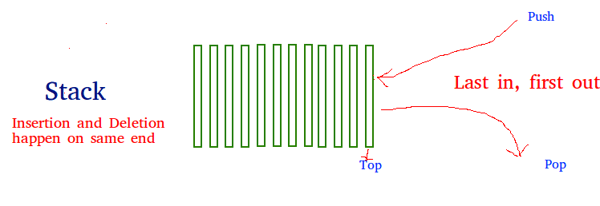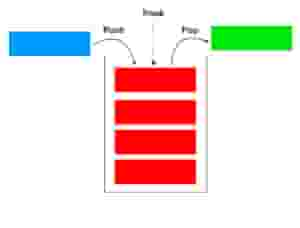Related Tags

python
community creator

# Different ways to implement stack in Python

Srija Chigurupati

## What is a stack?

A stack is a linear data structure in which all the insertion and deletion of data (i.e., values) are only done at one end rather than in the middle. Stacks can be implemented by using arrays of linear type.

The stack is mostly used when converting and evaluating expressions in Polish notations, i.e.,

• Infix
• Prefix
• Postfix## Functions

• empty() – Returns whether or not the stack is empty
• size() – Returns the size of the stack
• top() – Returns a reference to the top most element of the stack
• push(g) – Adds the element ‘g’ at the top of the stack
• pop() – Deletes the top most element of the stack

## The three principle operations in stack

• Individual items can be added and stored in a stack using a push operation.
• Objects can be retrieved using a pop operation, which removes an item from the stack.
• The peek function will display the topmost element from the stack. This operation will not remove the item from the stack like in the pop operation.## Implementing a Python =stack

• list
• collections.deque
• queue.LifoQueue

## Implementation using list

The built-in list structure that you probably frequently use in your programs can be used as a stack. Instead of .push(), you can use .append() to add new elements to the top of your stack while .pop() removes the elements in the LIFO order:

stack = []

# append() function to push
# element in the stack
stack.append('a')
stack.append('b')
stack.append('c')

print('Initial stack')
print(stack)

# pop() fucntion to pop
# element from stack in
# LIFO order
print('\nElements poped from stack:')
print(stack.pop())
print(stack.pop())
print(stack.pop())

print('\nStack after elements are poped:')
print(stack)

# uncommenting print(stack.pop())
# will cause an IndexError
# as the stack is now empty


## Implementation using collections.deque

Python stack can also be implemented using the deque class from the collections module. Deque is preferred over list in cases where we need to quickly append and pop operations from both the ends of the container. Deque provides an $O(1)$ time complexity for append and pop operations, while list provides a $O(n)$ time complexity. The same methods (append() and pop()) are used on deque as on list.

# Python program to
# demonstrate stack implementation
# using collections.deque
from collections import deque
stack = deque()
# append() function to push
# element in the stack
stack.append('a')
stack.append('b')
stack.append('c')
print('Initial stack:')
print(stack)
# pop() fucntion to pop
# element from stack in
# LIFO order
print('\nElements poped from stack:')
print(stack.pop())
print(stack.pop())
print(stack.pop())
print('\nStack after elements are poped:')
print(stack)
# uncommenting print(stack.pop())
# will cause an IndexError
# as the stack is now empty


## Implementation using queue module

The queue module also has a LIFO Queue that is basically a stack. Data is inserted into queue using the put() function and get() takes data out from the Queue. There are various functions available in this module:

• maxsize– Number of items allowed in the queue.
• empty() – Returns true if the queue is empty, and false if otherwise.
• full() – Returns true if there are maxsize items in the queue. If the queue was initialized with maxsize=0 (the default), then full() never returns True.
• get() – Removes and returns an item from the queue. If queue is empty, wait until an item is available.
• get_nowait() – Returns an item if one is immediately available, else it raises QueueEmpty. put(item) – Puts an item into the queue. If the queue is full, wait until a free slot is available before adding the item.
• put_nowait(item) – Puts an item into the queue without blocking.
• qsize() – Returns the number of items in the queue. If no free slot is immediately available, raise QueueFull.
# Python program to
# demonstrate stack implementation
# using queue module

from queue import LifoQueue

# Initializing a stack
stack = LifoQueue(maxsize = 3)

# qsize() show the number of elements
# in the stack
print(stack.qsize())

# put() function to push
# element in the stack
stack.put('a')
stack.put('b')
stack.put('c')

print("Full: ", stack.full())
print("Size: ", stack.qsize())

# get() fucntion to pop
# element from stack in
# LIFO order
print('\nElements poped from the stack')
print(stack.get())
print(stack.get())
print(stack.get())

print("\nEmpty: ", stack.empty())

RELATED TAGS

python
community creator

CONTRIBUTOR

Srija Chigurupati
RELATED COURSES

View all Courses

Keep Exploring

Learn in-demand tech skills in half the time